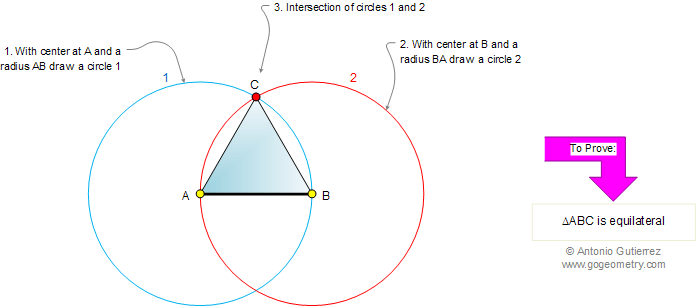Euclid's Elements Book I, Proposition 1: On a given finite line to construct an equilateral triangle.

Let AB be the given finite straight line. Thus it is required to construct an equilateral triangle on the straight line AB.ConstructionThe Elements: Books I-XIII
 Euclid's Elements is the oldest mathematical and geometric treatise consisting of 13 books written by Euclid in Alexandria c. 300 BC. It is a collection of definitions, postulates, axioms, 467 propositions (theorems and constructions), and mathematical proofs of the propositions.